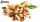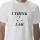# Simple equation 6

Solve equation with one variable:

X/2+X/3+X/4=X+4

Result

X =  48

#### Solution:

X/2+X/3+X/4=X+4

X = 48

X = 48

Calculated by our simple equation calculator.

Leave us a comment of example and its solution (i.e. if it is still somewhat unclear...):

Showing 0 comments:Be the first to comment!#### To solve this verbal math problem are needed these knowledge from mathematics:

Need help calculate sum, simplify or multiply fractions? Try our fraction calculator. Do you have a linear equation or system of equations and looking for its solution? Or do you have quadratic equation?

## Next similar examples:

1. Simple equation 5Solve equation with fractions: X × 3/8 = 1/2
2. Equation with xSolve the following equation: 2x- (8x + 1) - (x + 2) / 5 = 9
3. Equation with fractionsSolve equation: ? It is equation with fractions.
4. EqnSolve equation with fractions: 2x/3-50=40+x/4
5. Football match 4In a football match with the Italy lost 3 goals with Germans. Totally fell 5 goals in the match. Determine the number of goals of Italy and Germany.
6. Trees - orchardThe 3/5 trees are apple trees and 1/3 of the trees are cherries. The remaining 5 trees are pear trees. How many trees are in the orchard.
7. FractionsThree-quarters of an unknown number are 4/5. What is 5/6 of this unknown number?
8. Bag of peanutsJoe eat 1/3 of a bag of peanuts, mark eat 1/4 of the remaining in the bag of peanuts, Alvin eat 1/2 of the remaining bag of peanuts, peter eat 10 peanuts, there are 71 peanuts left. Hon many peanuts were in the bags?
9. Three monksThree medieval monks has task to copy 600 pages of the Bible. One rewrites in three days 1 page, second in 2 days 3 pages and a third in 4 days 2 sides. Calculate for how many days and what day the monks will have copied whole Bible when they begin Wednesd
10. Cleaning windowsCleaning company has to wash all the windows of the school. The first day washes one-sixth of the windows of the school, the next day three more windows than the first day and the remaining 18 windows washes on the third day. Calculate how many windows ha
11. UN 1If we add to an unknown number his quarter, we get 210. Identify unknown number.
12. One thirdIf 3/5 is 360, how much is 1/3?
13. Equation 20In given equation: 8/9-4/5=2/9+x, find x
14. Fifth of the numberThe fifth of the number is by 24 less than that number. What is the number?
15. Proof IWhen added to the product of two consecutive integers larger one, we get square larger one. Is this true or not?
16. Unknown numberIdentify unknown number which 1/5 is 40 greater than one tenth of that number.
17. Six yearsIn six years Jan will be twice as old as he was six years ago. How old is he?